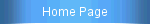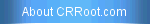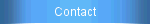Calculus Tutoring or High school classes for
Calculus, AP Calculus AB and AP Calculus BC.

Calculus Tutoring and High School Classes
offered. The textbook is Stewart 5e and is used
in High Schools and Colleges.Chapter 3 Solutions Odd Number Problems (6th e)
Chapter 3 Odd based Solutions
Chapter 2.1 The Tangent and Velocity Problems
Ch 2.1
Ch 2.1 Solutions
Chapter 2.3 Calculating Limits Using the Limit Laws
Ch 2.3
Ch 2.3 Solutions
Chapter 2.4 The Precise Definition of a Limit
Ch 2.4
Ch 2.4 Solutions
Chapter 2.5 Continuity
Ch 2.5
Ch 2.5 Solutions
Chapter 2.6 Limits at Infinity, Horizontal Limits
Ch 2.6
Ch 2.6 Solutions
Chapter 2.7 Tangents, Velocities, and other Rates of Change
Ch 2.7
Ch 2.7 Solutions
Chapter 2.8 Derivatives
Ch 2.8
Ch 2.8 Solutions
Chapter 2.9 The Derivative as a Function.
Ch 2.9
Ch 2.9 Solutions